# Chapter 4 Grammars and Parsing 1 Grammar Grammars

• Slides: 43Chapter 4 Grammars and Parsing 1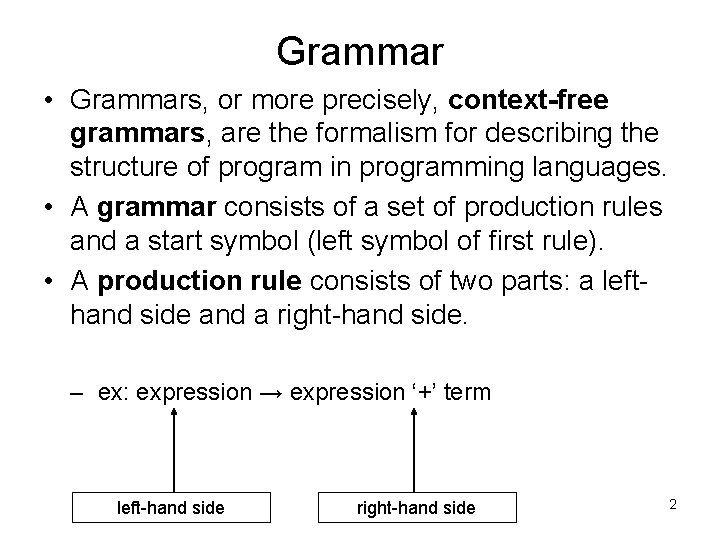Grammar • Grammars, or more precisely, context-free grammars, are the formalism for describing the structure of program in programming languages. • A grammar consists of a set of production rules and a start symbol (left symbol of first rule). • A production rule consists of two parts: a lefthand side and a right-hand side. – ex: expression → expression ‘+’ term left-hand side right-hand side 2Grammar (Cont. ) • The left-hand side is the name of the syntactic construct. • The right-hand side shows a possible form of the syntactic construct. • There are two possible forms (rules) derived by the name “expression”: expression → expression ‘+’ term (rule 1) expression → expression ‘-’ term (rule 2) 3Grammar (Cont. ) • The right-hand side of a production rule can contain two kinds of symbols: terminal and non-terminal. • A terminal symbol (or terminal) is an end point of the production process, also called token. Use lower-case letters such as a, b. • A non-terminal symbol (or non-terminal) must occur as the left-hand side of one or more production rules. Use upper-case letters such as A, B, S. • Non-terminal and terminal together are called grammar symbols. 4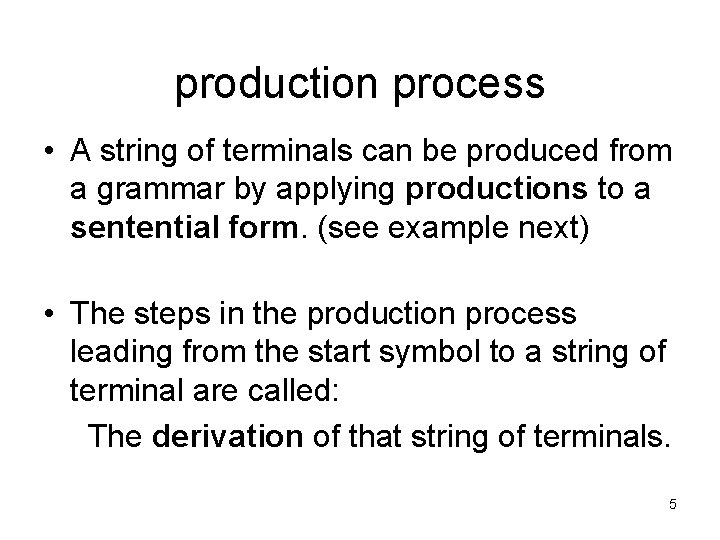production process • A string of terminals can be produced from a grammar by applying productions to a sentential form. (see example next) • The steps in the production process leading from the start symbol to a string of terminal are called: The derivation of that string of terminals. 5An example of production process • Grammar : – expression → ‘(‘ expression operator expression ‘)’ – expression → ‘ 1’ – operator → ‘+’ – operator → ‘*’ 6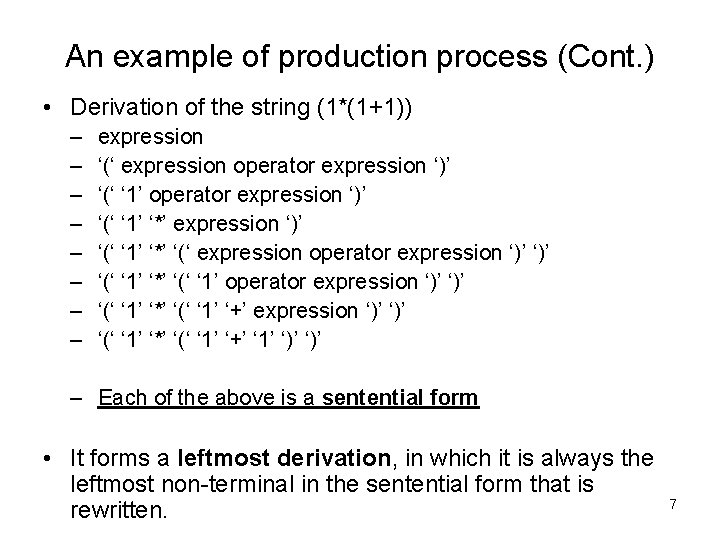An example of production process (Cont. ) • Derivation of the string (1*(1+1)) – – – – expression ‘(‘ expression operator expression ‘)’ ‘(‘ ‘ 1’ ‘*’ ‘(‘ expression operator expression ‘)’ ‘)’ ‘(‘ ‘ 1’ ‘*’ ‘(‘ ‘ 1’ ‘+’ expression ‘)’ ‘(‘ ‘ 1’ ‘*’ ‘(‘ ‘ 1’ ‘+’ ‘ 1’ ‘)’ – Each of the above is a sentential form • It forms a leftmost derivation, in which it is always the leftmost non-terminal in the sentential form that is rewritten. 7The definition of a grammar Context-free grammar (CFG) is defined by: (1) A finite terminal vocabulary Vt; this is the token set produced by the scanner. (2) A finite set of different, intermediate symbols, called the non-terminal vocabulary Vn. (3) A start symbol S Vn that starts all derivations. A start symbol is sometimes called a goal symbol. (4) P, a finite set of productions (sometimes called rewriting rules) of the form A → X 1…Xm, where A Vn, Xi Vn ∪ Vt, 1<=i<=m, m>=0 8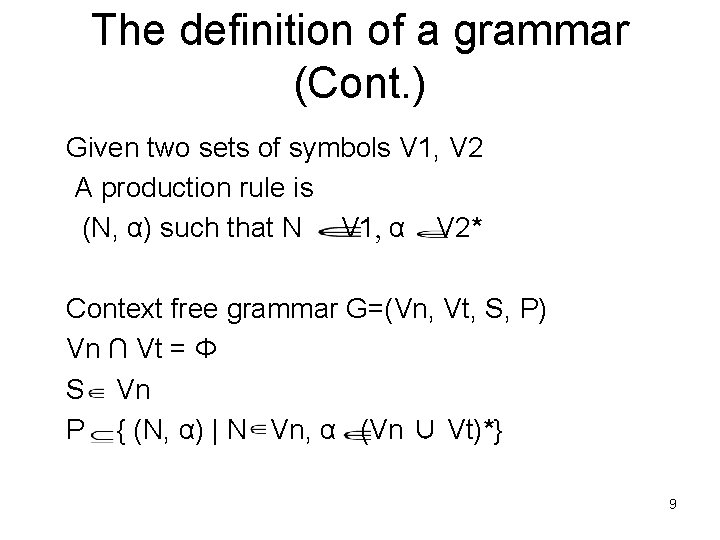The definition of a grammar (Cont. ) Given two sets of symbols V 1, V 2 A production rule is (N, α) such that N V 1, α V 2* Context free grammar G=(Vn, Vt, S, P) Vn ∩ Vt = Φ S Vn P { (N, α) | N Vn, α (Vn ∪ Vt)*} 9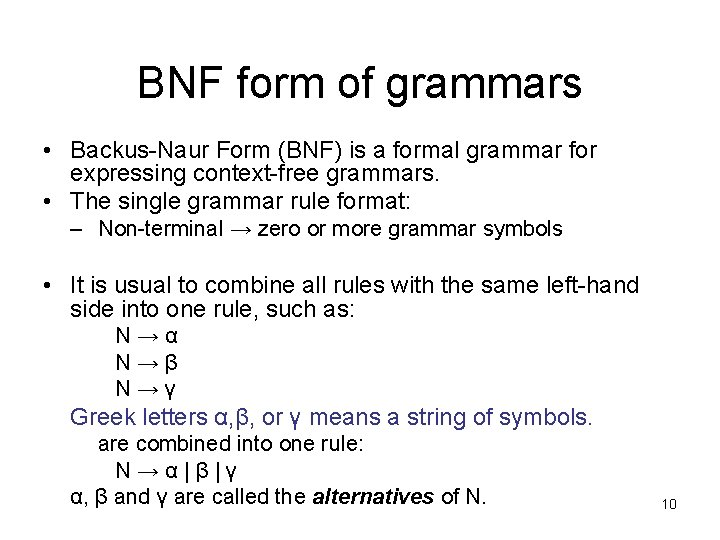BNF form of grammars • Backus-Naur Form (BNF) is a formal grammar for expressing context-free grammars. • The single grammar rule format: – Non-terminal → zero or more grammar symbols • It is usual to combine all rules with the same left-hand side into one rule, such as: N→α N→β N→γ Greek letters α, β, or γ means a string of symbols. are combined into one rule: N→α|β|γ α, β and γ are called the alternatives of N. 10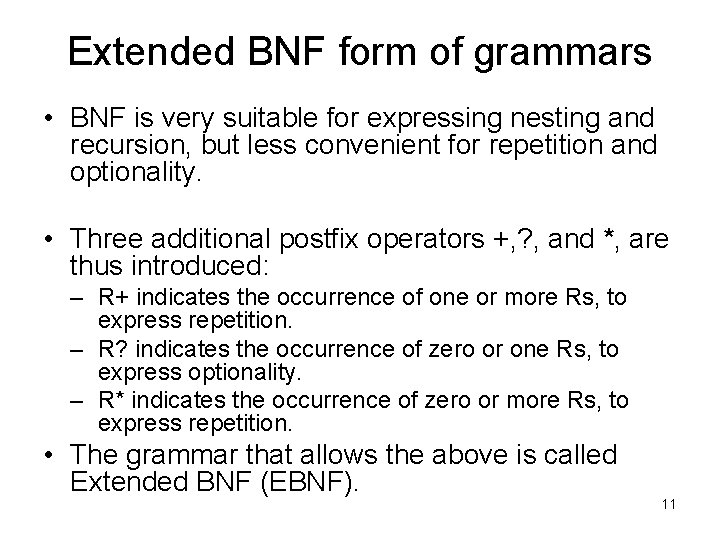Extended BNF form of grammars • BNF is very suitable for expressing nesting and recursion, but less convenient for repetition and optionality. • Three additional postfix operators +, ? , and *, are thus introduced: – R+ indicates the occurrence of one or more Rs, to express repetition. – R? indicates the occurrence of zero or one Rs, to express optionality. – R* indicates the occurrence of zero or more Rs, to express repetition. • The grammar that allows the above is called Extended BNF (EBNF). 11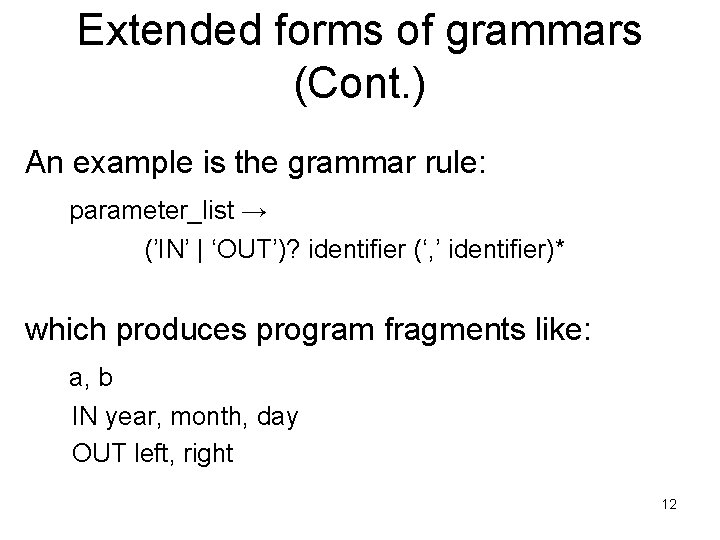Extended forms of grammars (Cont. ) An example is the grammar rule: parameter_list → (’IN’ | ‘OUT’)? identifier (‘, ’ identifier)* which produces program fragments like: a, b IN year, month, day OUT left, right 12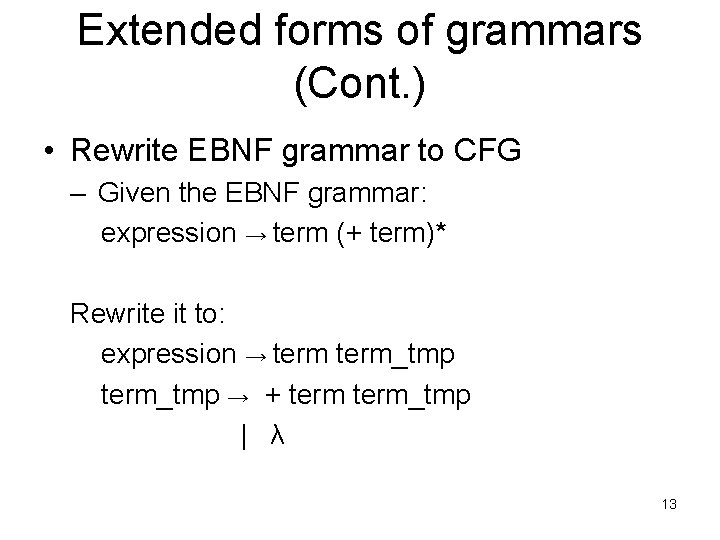Extended forms of grammars (Cont. ) • Rewrite EBNF grammar to CFG – Given the EBNF grammar: expression → term (+ term)* Rewrite it to: expression → term_tmp → + term_tmp | λ 13Properties of grammars • A non-terminal N is left-recursive if, starting with a sentential form N, we can produce another sentential form starting with N. – ex: expression → expression ‘+’ factor | factor • right-recursion also exists, but is less important. – ex: expression → term ‘+’ expression 14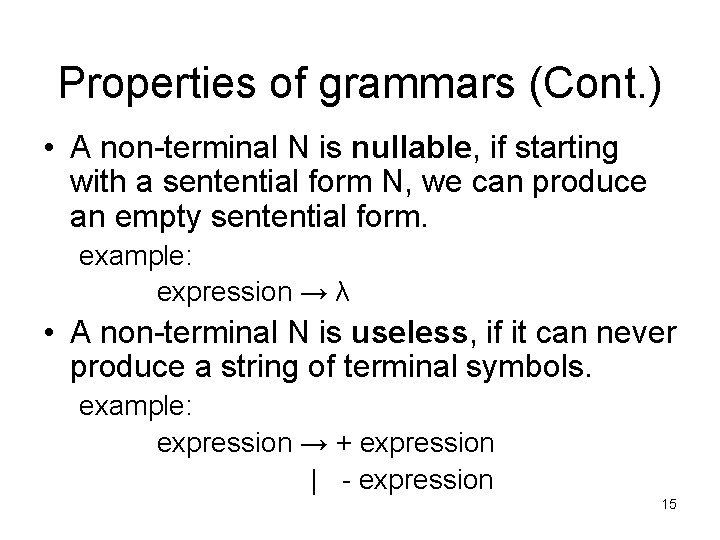Properties of grammars (Cont. ) • A non-terminal N is nullable, if starting with a sentential form N, we can produce an empty sentential form. example: expression → λ • A non-terminal N is useless, if it can never produce a string of terminal symbols. example: expression → + expression | - expression 15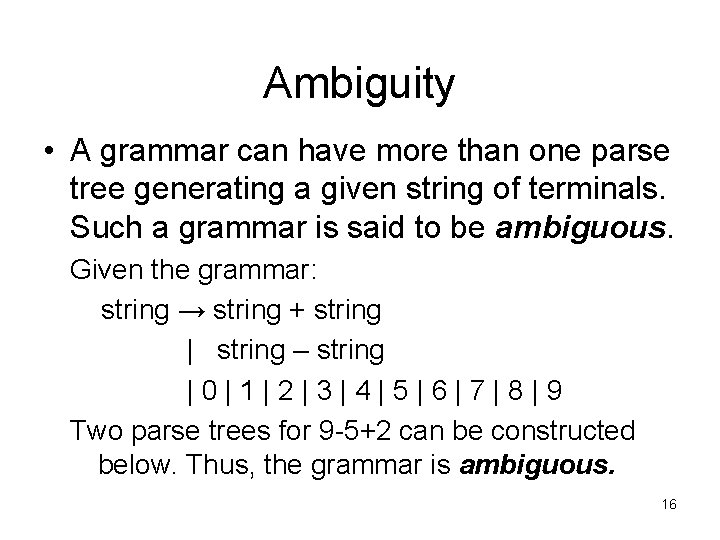Ambiguity • A grammar can have more than one parse tree generating a given string of terminals. Such a grammar is said to be ambiguous. Given the grammar: string → string + string | string – string |0|1|2|3|4|5|6|7|8|9 Two parse trees for 9 -5+2 can be constructed below. Thus, the grammar is ambiguous. 16Ambiguity string + string - string 9 5 string 2 string - string 9 string + string 5 2 17Associativity of operators • Left-associativity: 9+5+2 is equivalent to 9+5+2 • Given the grammar: – list → list + digit – | list – digit – | digit – digit → 0 | 1 | 2 | 3 | 4 | 5 | 6 | 7 | 8 | 9 18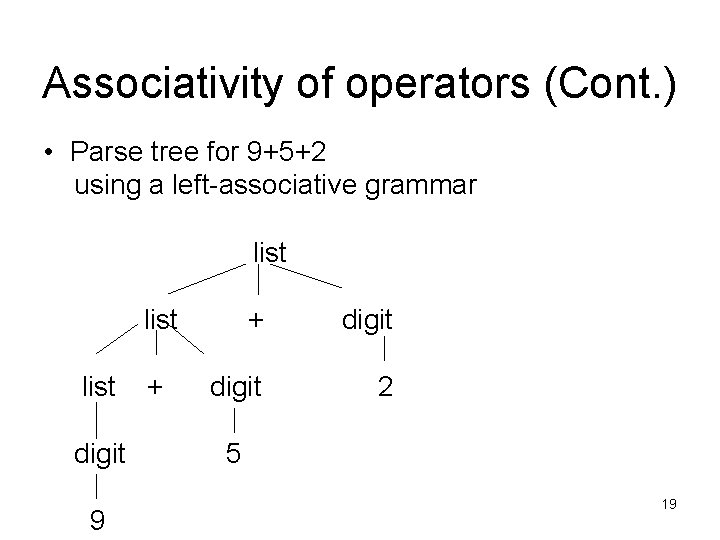Associativity of operators (Cont. ) • Parse tree for 9+5+2 using a left-associative grammar list digit 9 + + digit 2 5 19Associativity of operators (Cont. ) • Right-associativity: expression a=b=c is treated in the same way as the expression a=b=c • Given the grammar: – right → letter = right – | letter – letter → a | b | … | z 20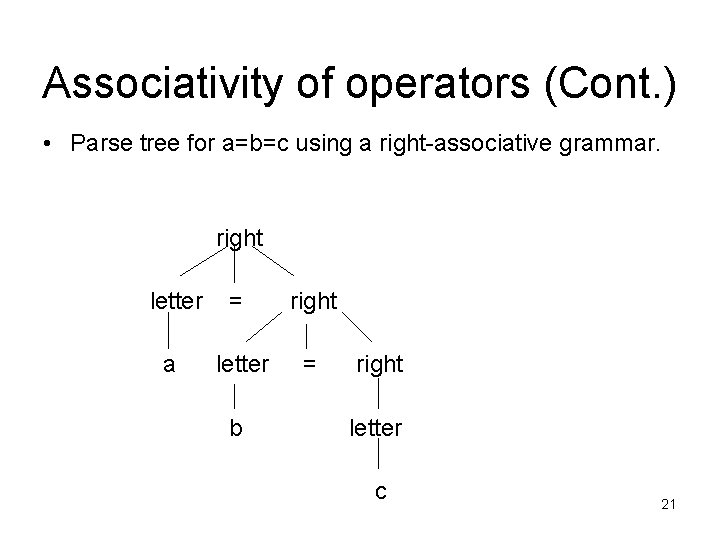Associativity of operators (Cont. ) • Parse tree for a=b=c using a right-associative grammar. right letter = right a letter = b right letter c 21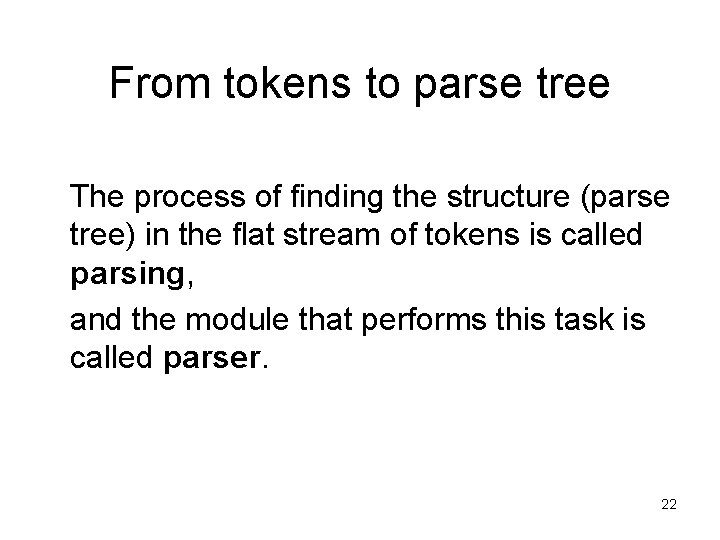From tokens to parse tree The process of finding the structure (parse tree) in the flat stream of tokens is called parsing, and the module that performs this task is called parser. 22Parsing methods The way to construct the parse tree: – Leaf nodes are labeled with terminals and inner nodes are labeled with non-terminals. – The top node is labeled with the start symbol. – The children of an inner node labeled N correspond to the members of an alternative of N, in the same order as they occur in that alternative. – The terminals labeling the leaf nodes correspond to the sequence of tokens, in the same order as they occur in the input. 23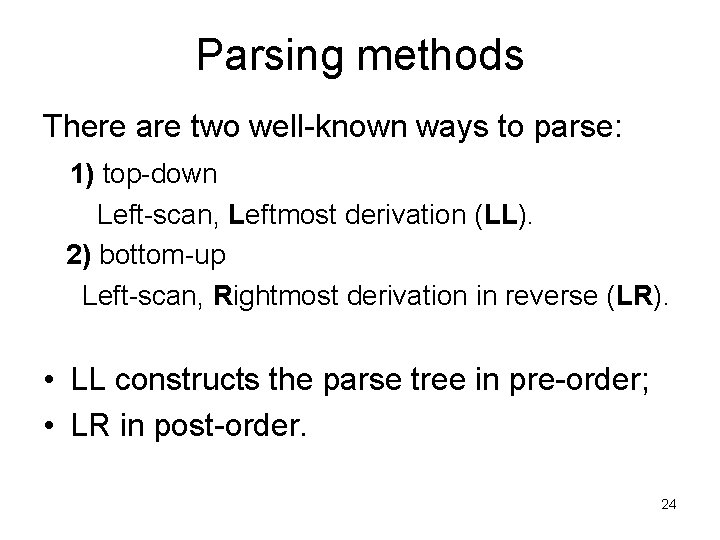Parsing methods There are two well-known ways to parse: 1) top-down Left-scan, Leftmost derivation (LL). 2) bottom-up Left-scan, Rightmost derivation in reverse (LR). • LL constructs the parse tree in pre-order; • LR in post-order. 24Pre-order vs. post-order traversal • When traversing a node N in pre-order, the process first visits the node N and then traverses N’s subtrees in left-to-right order. • When traversing a node N in post-order, the process first traverses N’s subtrees in left-to-right order and then visits the node N. 25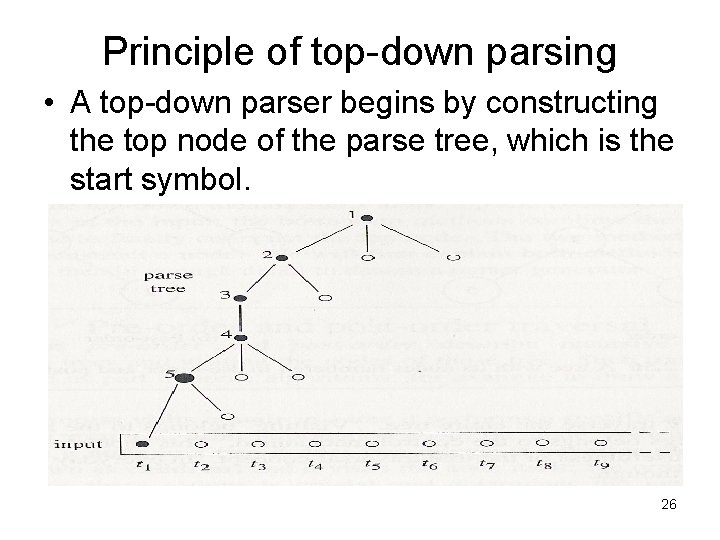Principle of top-down parsing • A top-down parser begins by constructing the top node of the parse tree, which is the start symbol. 26Principles of bottom-up parsing • The bottom-up parsing method constructs the nodes in the parse tree in post-order. 27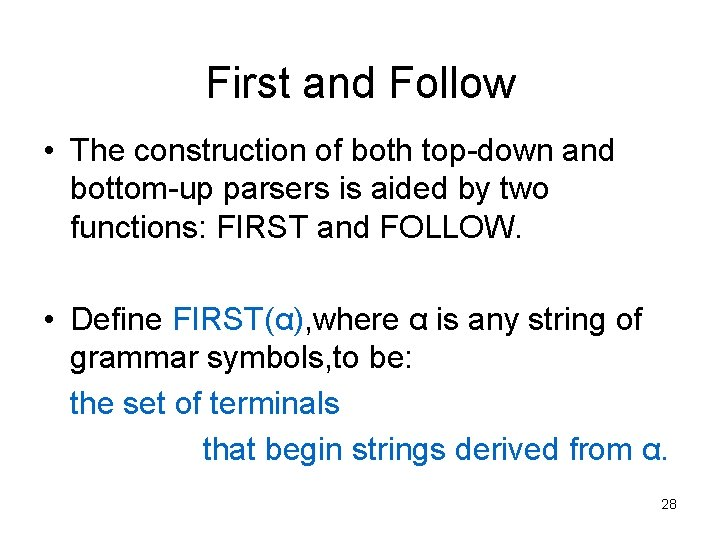First and Follow • The construction of both top-down and bottom-up parsers is aided by two functions: FIRST and FOLLOW. • Define FIRST(α), where α is any string of grammar symbols, to be: the set of terminals that begin strings derived from α. 28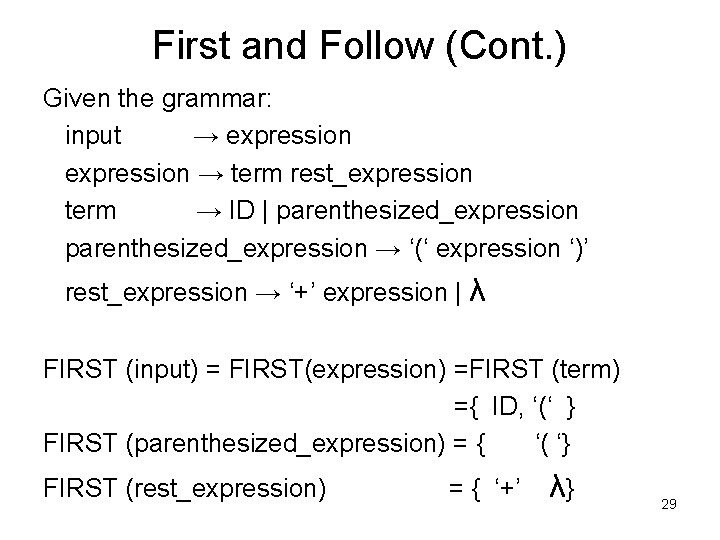First and Follow (Cont. ) Given the grammar: input → expression → term rest_expression term → ID | parenthesized_expression → ‘(‘ expression ‘)’ rest_expression → ‘+’ expression | λ FIRST (input) = FIRST(expression) =FIRST (term) ={ ID, ‘(‘ } FIRST (parenthesized_expression) = { ‘( ‘} FIRST (rest_expression) = { ‘+’ λ} 29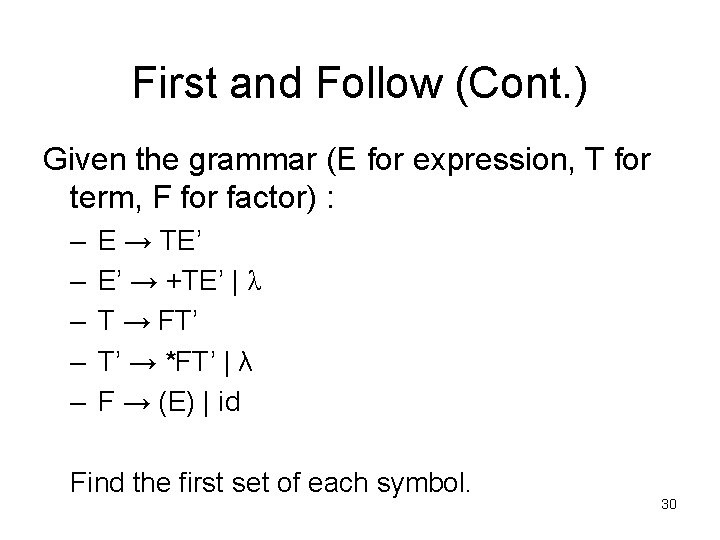First and Follow (Cont. ) Given the grammar (E for expression, T for term, F for factor) : – – – E → TE’ E’ → +TE’ | λ T → FT’ T’ → *FT’ | λ F → (E) | id Find the first set of each symbol. 30First and Follow (Cont. ) Answer: FIRST(F) = FIRST(T) = FIRST(E) = {(, id } FIRST(E’) = {+, λ} FIRST(T’) = {*, λ} 31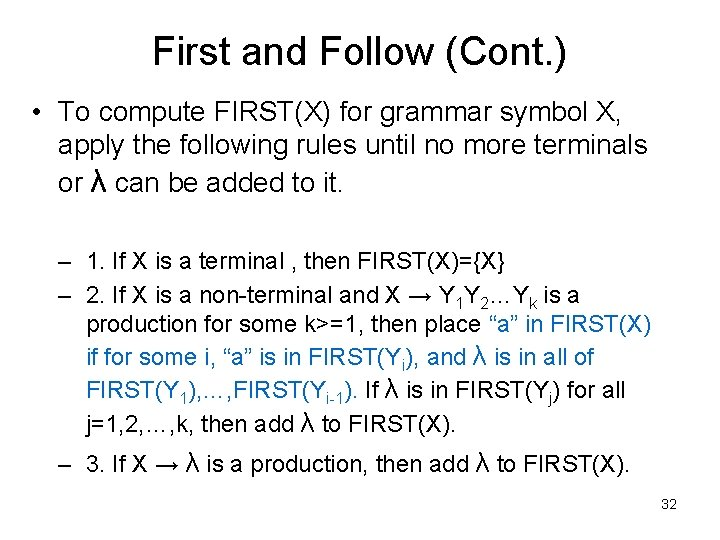First and Follow (Cont. ) • To compute FIRST(X) for grammar symbol X, apply the following rules until no more terminals or λ can be added to it. – 1. If X is a terminal , then FIRST(X)={X} – 2. If X is a non-terminal and X → Y 1 Y 2…Yk is a production for some k>=1, then place “a” in FIRST(X) if for some i, “a” is in FIRST(Yi), and λ is in all of FIRST(Y 1), …, FIRST(Yi-1). If λ is in FIRST(Yj) for all j=1, 2, …, k, then add λ to FIRST(X). – 3. If X → λ is a production, then add λ to FIRST(X). 32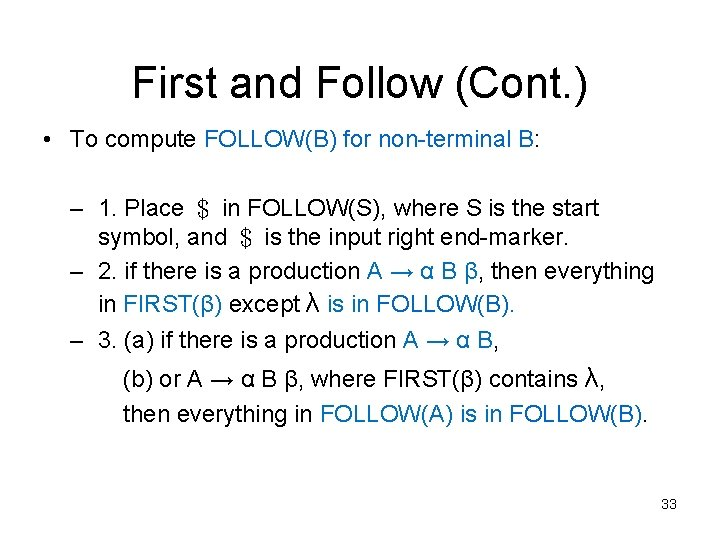First and Follow (Cont. ) • To compute FOLLOW(B) for non-terminal B: – 1. Place ＄ in FOLLOW(S), where S is the start symbol, and ＄ is the input right end-marker. – 2. if there is a production A → α B β, then everything in FIRST(β) except λ is in FOLLOW(B). – 3. (a) if there is a production A → α B, (b) or A → α B β, where FIRST(β) contains λ, then everything in FOLLOW(A) is in FOLLOW(B). 33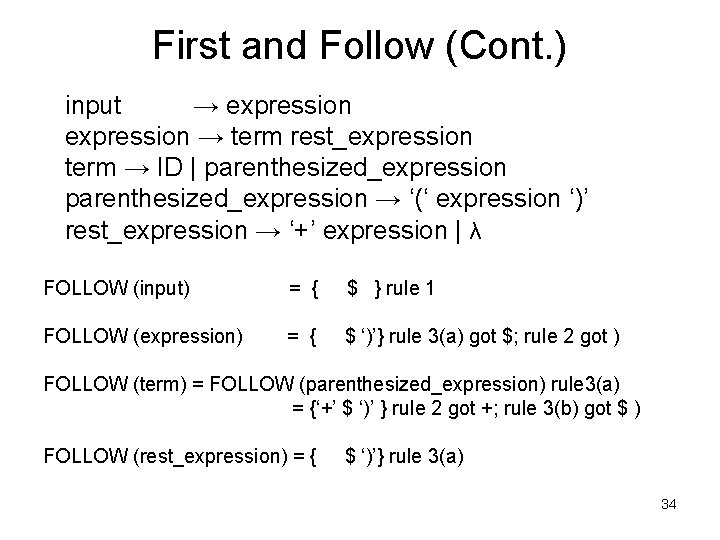First and Follow (Cont. ) input → expression → term rest_expression term → ID | parenthesized_expression → ‘(‘ expression ‘)’ rest_expression → ‘+’ expression | λ FOLLOW (input) = { \$ } rule 1 FOLLOW (expression) = { \$ ‘)’} rule 3(a) got \$; rule 2 got ) FOLLOW (term) = FOLLOW (parenthesized_expression) rule 3(a) = {‘+’ \$ ‘)’ } rule 2 got +; rule 3(b) got \$ ) FOLLOW (rest_expression) = { \$ ‘)’} rule 3(a) 34First and Follow (Cont. ) • For example, given the grammar : – – – E → TE’ E’ → +TE’ | λ T → FT’ T’ → *FT’ | λ F → (E) | id Find the follow set of each symbol. 35First and Follow (Cont. ) Answers: FOLLOW(E) = FOLLOW(E’) = { ）, ＄} FOLLOW(T) = FOLLOW(T’) = { +, ）, ＄} FOLLOW(F) = {＊, + , ）, ＄} 36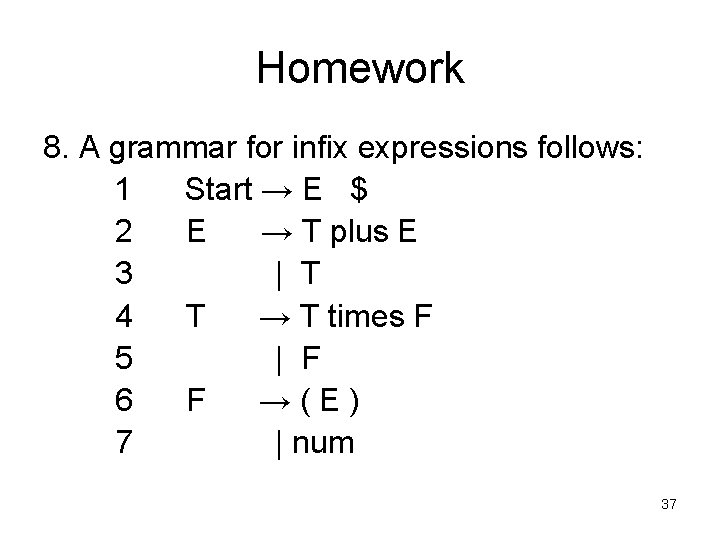Homework 8. A grammar for infix expressions follows: 1 Start → E \$ 2 E → T plus E 3 | T 4 T → T times F 5 | F 6 F →(E) 7 | num 37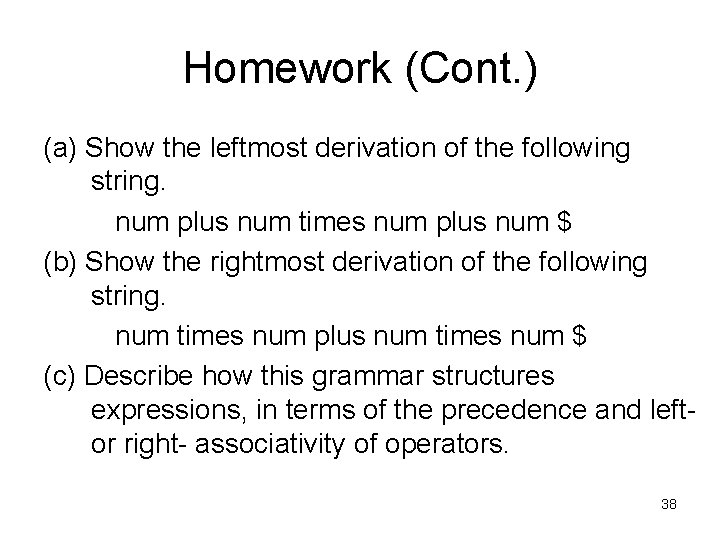Homework (Cont. ) (a) Show the leftmost derivation of the following string. num plus num times num plus num \$ (b) Show the rightmost derivation of the following string. num times num plus num times num \$ (c) Describe how this grammar structures expressions, in terms of the precedence and leftor right- associativity of operators. 38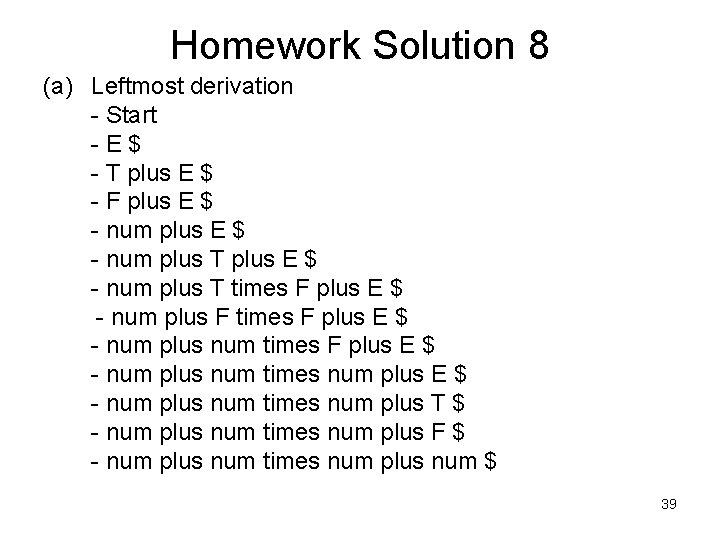Homework Solution 8 (a) Leftmost derivation - Start -E\$ - T plus E \$ - F plus E \$ - num plus T times F plus E \$ - num plus F times F plus E \$ - num plus num times num plus T \$ - num plus num times num plus F \$ - num plus num times num plus num \$ 39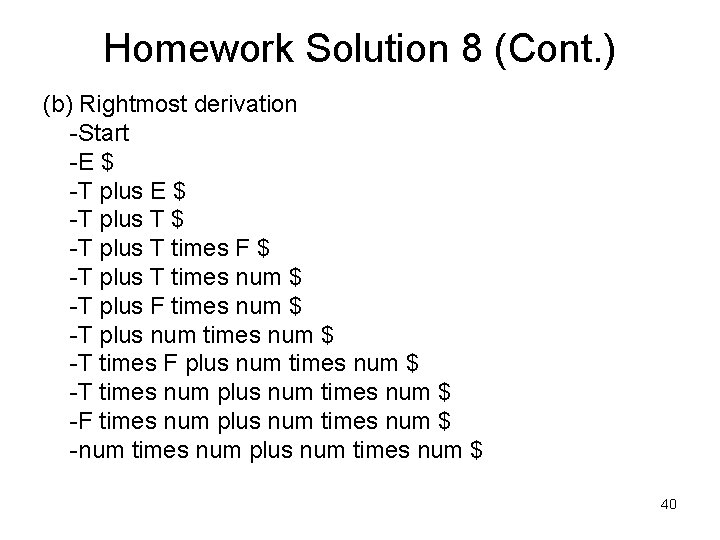Homework Solution 8 (Cont. ) (b) Rightmost derivation -Start -E \$ -T plus T times F \$ -T plus T times num \$ -T plus F times num \$ -T plus num times num \$ -T times F plus num times num \$ -T times num plus num times num \$ -F times num plus num times num \$ -num times num plus num times num \$ 40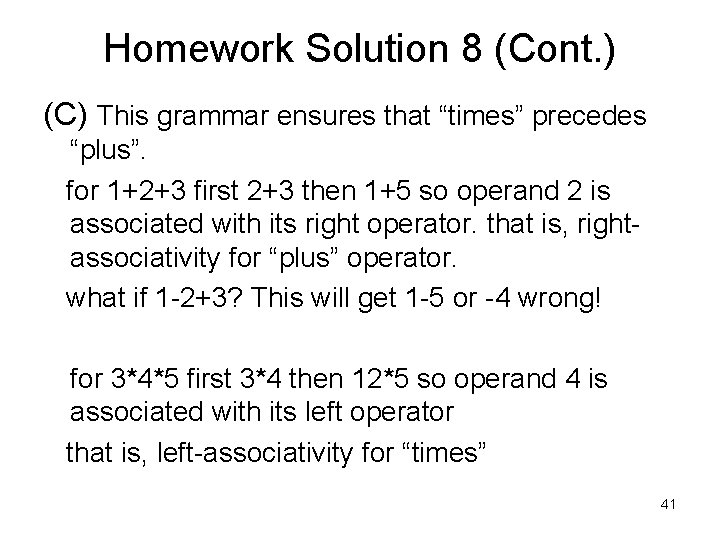Homework Solution 8 (Cont. ) (C) This grammar ensures that “times” precedes “plus”. for 1+2+3 first 2+3 then 1+5 so operand 2 is associated with its right operator. that is, rightassociativity for “plus” operator. what if 1 -2+3? This will get 1 -5 or -4 wrong! for 3*4*5 first 3*4 then 12*5 so operand 4 is associated with its left operator that is, left-associativity for “times” 41Homework (Cont. ) 11 Compute First and Follow sets for the nonterminals of the following grammar 1 2 3 4 5 6 S→a. Se | B B→b. Be | C C→c. Ce | d 42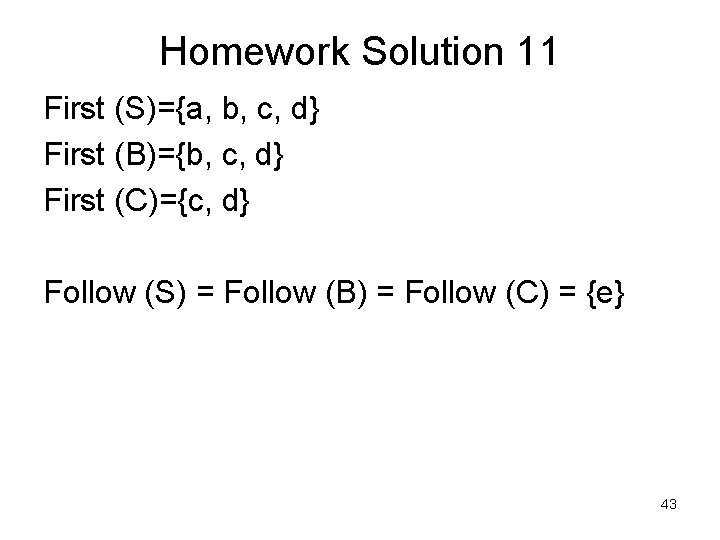Homework Solution 11 First (S)={a, b, c, d} First (B)={b, c, d} First (C)={c, d} Follow (S) = Follow (B) = Follow (C) = {e} 43